# Determining the Viscoelastic Behavior of Agarose Gel

Agarose hydrogels are rapidly becoming a popular option for an implantable scaffold material due to their biocompatibility, native tissue-like viscoelastic mechanical properties and ease of casting into complex shapes and sizes. Hydrogel implants have been used in applications where structural integrity is necessary, such as cartilage and skin. With a key functional role of cartilage being force dampening, any potential substitute must be capable of sustaining high impact loading. This series of experiments uses the ElectroForce® 3200 test instrument to characterize the dynamic mechanical properties of agarose gels at high frequencies.

## Determining Dynamic Modulus and Tan Delta of Agarose Hydrogels

Dr. Murakami and Dr. Sawae, lead researchers at the Kyushu Institute of Technology in Japan, were interested in investigating the mechanical behavior (dynamic modulus and tan delta) of low concentration agarose gels at high frequencies.To accomplish this, the ElectroForce 3200 test instrument, in combination with WinTest® software, was used to perform DMA (Dynamic Mechanical Analysis) experiments at frequencies that ranged from 0.1 Hz to 100 Hz at different mean displacements.

## Testing Procedure

1% and 2% (wt.) agarose gels were produced from agarose type VII powder (Sigma-Aldrich) using a standard gelling protocol supplied by Dr. Murakami. Test specimens, cut from the bulk gels, were approximately 18 mm in diameter and 2.5 mm in height. 50 mm diameter solid platens were used to secure the sample. An ElectroForce 3200 test instrument equipped with a 1000 g load cell was used in these experiments. DMA was set up as a frequency sweep from 0.1 Hz to 100 Hz at a range of mean displacements.

These mean amplitudes, as measured by stretch ratio, ranged from 0.99 to 0.70, where the stretch ratio, λ, is defined as λ= ℓ / L0, where l is the loaded height and L0 is the unloaded height. The displacement amplitude was ±0.5% of the unloaded height.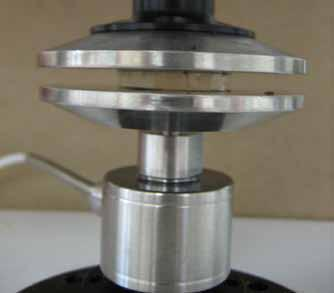## DMA Results

The DMA software immediately produces usable results at the end of the experiments. One example of this is the complex modulus shown in Figure 1. As the frequency is increased, complex modulus asymptotically increases. In addition, the software generates the two components: storage and loss modulus.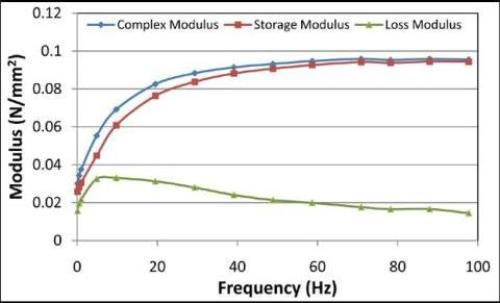Figure 1. 1% Agarose Modulus at λ = 0.9

The contribution to the complex modulus at lower frequencies is approximately equal, while the storage modulus dominates at higher frequencies. This is an indication that less energy is dissipated at higher frequencies.

While this figure provides insight into the material behavior at λ = 0.9, there is a wide range of other loading scenarios this material can experience. Figures 2 and 3 quantify the material responses at different mean stretch ratios. Tan delta, an indication of the phase shift between displacement and load, is shown for the 1% agarose gel. There is a much larger phase shift at lower frequencies. This effect can be understood in Figure 1 as the loss modulus does not greatly contribute to complex modulus at higher frequencies.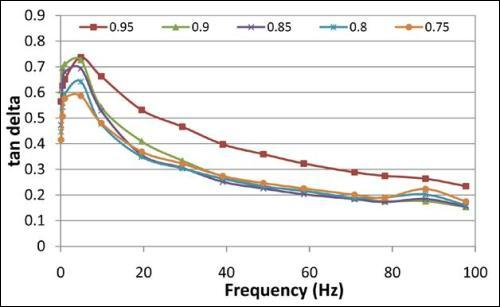Figure 2. 1% Agarose, Tan Delta at Different Mean λ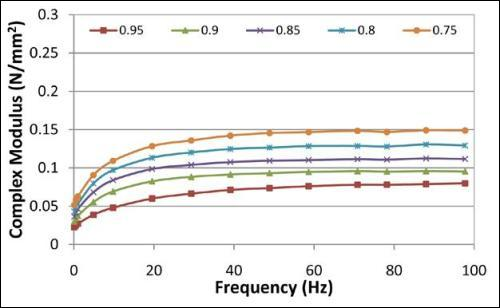Figure 3. 1% Agarose, Modulus at Different Mean λ

Figure 3, complex modulus at different λ, provides more insight into the material behavior. It can also be seen that as the mean stretch ratio is decreased (i.e., the material is more compressed), the modulus of that material is significantly increased. Analyzing this graph along with tan delta information from Figure 2 shows that the material behaves significantly differently depending upon pre-stretch. As the stretch ratio is decreased, the contribution by the loss modulus to the complex modulus is substantially reduced. Therefore, as the material is initially more compressed, the behavior resembles a more purely elastic material.

Similar results can be seen for the 2% agarose gel with different magnitudes. Tan delta for the 2% agarose gel is less than 1% gels at lower frequencies (Figure 4). Additionally, the complex modulus of the 2% agarose gel, shown in Figure 5, is greater than the modulus for the 1% agarose gel.

Plotting this data in three dimensional space leads to further understanding of experimental results, as shown in Figure 6. This figure displays the complex modulus of a 1% agarose gel as a function of both the frequency and mean stretch ratio. A surface can be fit to this data using mathematical models which would lead to predictive capabilities. Similar graphs can be developed displaying tan delta as a function of both mean stretch ratio and frequency.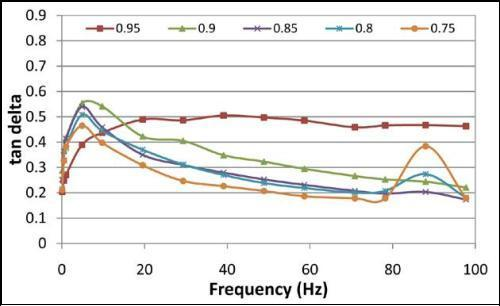Figure 4. 2% Agarose, Tan Delta at Different Mean λ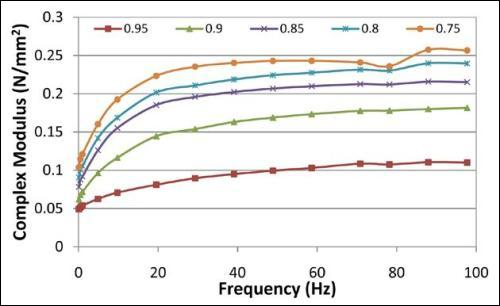Figure 5. 2% Agarose, Modulus at a Different Mean λ

Incorporating tan delta into the model would further strengthen the predictive capabilities of the model. With this knowledge of material behavior, scaffold design parameters, and clinical application of agarose gels can be understood prior to development.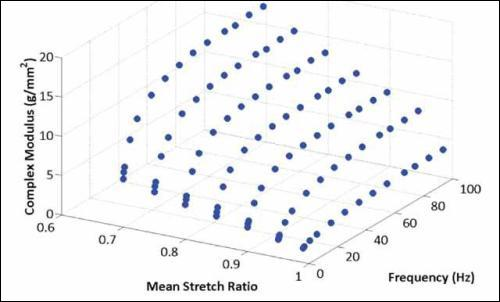Figure 6. 1% Agarose, Complex Modulus

## Summary

This series of experiments demonstrates that the ElectroForce® 3200 test instrument is capable of operating at the desired high frequencies while measuring small magnitude loads with accuracy and precision. With a variety of options for load cells and platens, the ElectroForce 3200 is well suited for use in testing weak viscoelastic materials.This information has been sourced, reviewed and adapted from materials provided by TA Instruments.

## Citations

• APA

TA Instruments. (2020, April 28). Determining the Viscoelastic Behavior of Agarose Gel. AZoM. Retrieved on October 25, 2020 from https://www.azom.com/article.aspx?ArticleID=5351.

• MLA

TA Instruments. "Determining the Viscoelastic Behavior of Agarose Gel". AZoM. 25 October 2020. <https://www.azom.com/article.aspx?ArticleID=5351>.

• Chicago

TA Instruments. "Determining the Viscoelastic Behavior of Agarose Gel". AZoM. https://www.azom.com/article.aspx?ArticleID=5351. (accessed October 25, 2020).

• Harvard

TA Instruments. 2020. Determining the Viscoelastic Behavior of Agarose Gel. AZoM, viewed 25 October 2020, https://www.azom.com/article.aspx?ArticleID=5351.